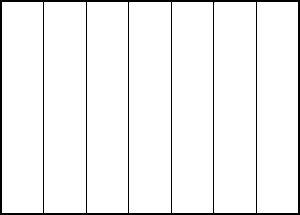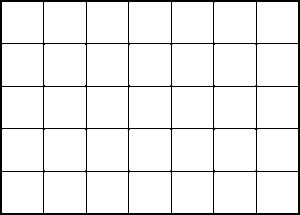# The Rectangle Area Formula—How Does Your Curriculum Introduce It?

Standards co-author Jason Zimba unpacks the rectangle area formula and highlights problems with the way textbooks sometimes introduce the formula to students.

Editor’s Note: This blog post originally appeared on Jason Zimba’s personal blog on August 10, 2016.

Has a student ever asked you why multiplying length by width gives the area of a rectangle? In this blog post, Standards co-author Jason Zimba describes the area formula on a conceptual level and highlights some weaknesses in the ways textbooks introduce the formula.

In the rectangle area formula A = × W,

• A is the number of unit squares needed to tile the rectangle
• L is the number of length units when you measure one side of the rectangle
• W is the number of length units when you measure an adjacent side of the rectangle.

The reason the formula works has to do with what the × symbol means.

Today let’s confine our analysis of the formula to whole numbers, because I’m thinking about the formula today from the perspective of a young student who hasn’t yet absorbed fractional quantities or fraction operations. For whole numbers, the simple interpretation of m × n is that it stands for the number of things in m groups of n things each.

In the context of a rectangle with, say, length 7 units and width 5 units, what are the groups, and what are the things?

If one side of the rectangle consists of 7 length units, then we can draw lines to divide the rectangle equally into 7 strips. There are as many strips in the rectangle as there are length units in the side.We might have done the same thing on either of the two adjacent sides, which would have resulted in the number of strips being 5.If we draw both sets of lines, then each of the strips gets divided into squares (question: why perfect squares?). For example, the 7 strips would turn into 7 groups of 5 squares each. Remembering what × means, the total number of squares must then equal 7 × 5.This argument is pretty obviously sound for any rectangle whatsoever with whole-number side lengths, so we can say that for any such rectangle:

The number of unit squares needed to tile a rectangle equals the number of length units that fit along one side multiplied by the number of length units that fit along an adjacent side.

This is what is meant by “Area equals length times width.”

Some drawbacks I have seen in the way some math textbooks teach A = L × W:

• Doing a poor job establishing what m × n means altogether. Then the book has no chance of doing a proper job with area, or with anything else that relies on multiplication.
• Doing a poor job with basic measurement concepts. Then it isn’t clear what a length measurement means, or what an area measurement means. You can’t relate two ideas you don’t grasp individually.
• Being unclear about the difference between area and length. An example of this would be blurring the distinction between iterations of length units along the boundary and iterations of area units just inside the boundary. One book says, “The number of squares in one row is the same as the length in unit squares of the rectangle.” What is an object’s “length in unit squares”? Unit squares are area units, not length units. A correct way to say it would be, “The number of squares in one row is the same as the number of length units in the edge of the rectangle.” Maybe it sounds convoluted, but in mathematics, words mean things; you have to combine the words into statements that are true, or at least not hostile to the distinction at hand.
• Taking too long to orchestrate the reasoning involved.

#### Tags:

About the Author: Jason Zimba was a lead writer of the Common Core State Standards for Mathematics and is a Founding Partner of Student Achievement Partners. He holds a B.A. from Williams College with a double major in mathematics and astrophysics; an M.Sc. by research in mathematics from the University of Oxford; and a Ph.D. in mathematical physics from the University of California at Berkeley. As a researcher, Dr. Zimba’s work spanned a range of fields, including astronomy, astrophysics theoretical physics, philosophy of science, and pure mathematics. His academic awards include a Rhodes scholarship and a Majorana Prize for theoretical physics. As an educator, he has taught physics, mathematics, and other subjects to college students, university physics and engineering majors, adult prison inmates, disadvantaged high school students, and children of non-English speaking immigrants.